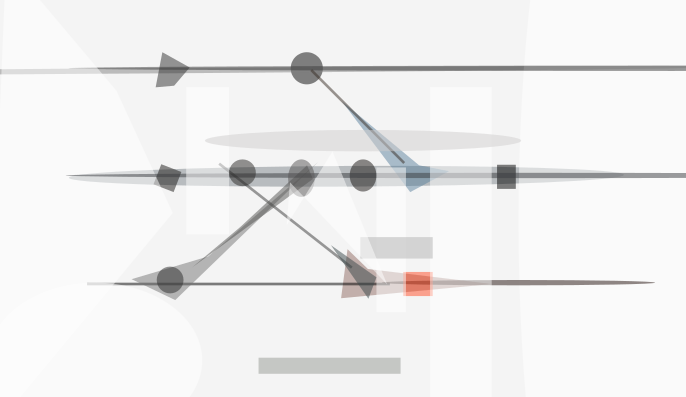# 逻辑时钟 - 如何刻画分布式中的事件顺序

• 为什么需要逻辑时钟 – 物理时钟是否可行？
• 相对论是带来了什么启示 – 事件的相对性
• 最早期的逻辑时钟算法什么特点 – Lamport逻辑时钟
• 后续改进的算法有哪些 – 「向量时钟」和「版本向量」等等

### 如何确定分布式系统中事件的发生顺序？ ¶

1. 首先，进程A中发生了一个事件$a$，并把这个事件消息同步给另外两个进程B和C。
2. B收到消息后，发生了一个事件$b$，并把这个事件消息同步给进程C。
3. 但是由于无法确定的网络延迟原因，导致进程A发出的消息到达C晚于进程B发出的消息到达C，这样 进程C的视角上，最终看到的事件顺序是 $b, a$，但是这与事实是相悖的。图1 - 由于网络延迟， 进程$B$发出的消息晚于进程$A$发出的消息到达进程$C$

1. 假设朋友圈有三个数据中心，分别在北京、维也纳和纽约。
2. 北京小明在朋友圈中发了一张风景图，并问到「猜猜这里是哪里?」，这条消息被扩散到其他数据中心。
3. 维也纳的小红看到这个消息后，回复说她知道。这条回复也被扩散到其他数据中心。
4. 但是由于无法确定的网络延迟的原因，导致纽约的数据中心先收到小红的回复，而后收到了原始的提问消息。 这样，导致最终小李看到的问答顺序是不符合问答的因果一致性的。

### 全局物理时钟 ¶

If you have one clock, you know what the time is. If you have two, you are not sure.

1. 仍然是由于网络延迟的不确定，我们无法通过网络同步时间来获取一个全局一致的物理时钟。
2. 现实中的多个时钟，即使时间已经调成一致，但是由于日积月累的计时速率的差异，会导致时钟漂移而显示不同的时间。

### 全序和偏序 ¶

1. 自反性： 对$S$中任意的元素$a$，都有 $a\le a$.
2. 反对称性： 如果对于$S$中的两个元素 $a$ 和 $b$， $a\le b$ 且 $b\le a$，那么 $a=b$
3. 传递性： 如果对于$S$中的三个元素，有$a\le b$ 且 $b\le c$， 那么 $a \le c$

1. 完全性： 对于$S$中的任意$a$ $b$元素，必然有 $a \le b$ 或 $b \le a$.

1. 自然数集合中的比较大小的关系，就是一种全序关系。
2. 集合之间的包含关系，则是一种偏序关系（集合之间可以有包含关系，也可以没有包含关系）。
3. 复数之间的大小关系，是一种偏序关系，而复数的模的大小关系，则是一种全序关系。图5 - $S1$描述了全序关系； $S2$描述了偏序关系

### 事件先后的相对性 ¶

Special relativity teaches us that there is no invariant total ordering of events in space-time; different observers can disagree about which of two events happened first. There is only a partial order in which an event e1 precedes an event e2 iff e1 can causally affect e2.

Lamport受相对论中事件顺序的相对性的启发，创造了Lamport Logical Clocks

• 在物理时空中，信息是通过光速传播的，而在分布式系统中，信息是通过网络传播的。
• 在物理时空中，不同参照系下的观察者可能对于事件顺序无法达成一致， 而在分布式系统中，由于全局物理时钟无法实现，不存在进程拥有全局视角。 而如果进程间的事件没有因果关系，那么就无法达成顺序上的一致性。
• 在物理时空中，由于光速限制，观察者在观察到事件$A$的时候，才确定了事件$B$和$A$的因果关系。 那么在分布式系统中，我们同样可以通过消息传递来创造因果关系。

• 红色的点表示自发性事件。 黑色的表示『观察到其他进程事件』而发生的事件。
• 横向黑色实线代表物理时钟。
• 带箭头的线表示进程中一个事件发生时，向另外一个进程传播这个事件。图8 - 示例： $S_{1}$和$S_{2}$虽然在物理时序上不一样，但是在各个进程的视角上推导出来却是一样的

### 逻辑时钟(Lamport’s timestamp) ¶

1. 进程内自发的事件（如下图中的红色标记的事件）。
2. 发送一个消息，是一个事件（如下图中的蓝色标记的事件）。
3. 接收一个消息，是一个事件 （如下图中的黑灰色标记的事件）。

if $e_{i}$ causes $e_{j}$, then $e_{i}$ must happen before $e_{j}$ – Logical Time

1. 如果 $a$ 和 $b$ 是同一个进程内的事件，并且 $a$ 在 $b$ 之前发生，则 $a \rightarrow b$。
2. 如果事件 $a$ 是「发送了一个消息」，而事件 $b$ 是接收了这个消息，则 $a \rightarrow b$。
3. 如果 $a \rightarrow b$ 并且 $b \rightarrow c$ ，那么 $a \rightarrow c$ （即传递性）。

• $a \rightarrow b \rightarrow c \rightarrow d$
• $e \rightarrow c \rightarrow d$
• $a \parallel e$
• $b \parallel e$

Lamport的时钟算法

1. 每个进程 $P_{i}$ 内维护本地一个计数器 $C_{i}$ ，初始为$0$.
2. 每次执行一个事件，计数器 $C_{i}$ 自增 （假设自增量为$1$）.
3. 进程 $P_{i}$ 发消息给进程 $P_{j}$ 时，需要在消息上附带自己的计数器 $C_{i}$.
4. 当进程 $P_{j}$ 接收到消息时，更新自己的计数器 $C_{j}=Max(C_{i}, C_{j})+1$

1. 假设 $a$ 和 $b$ 发生在同一个进程内，显然 $C_{a} < C_{b}$.
2. 假设 $a$ 和 $b$ 分别处在不同的进程内，如 $P_{a}$ 和 $P_{b}$，

根据事件先后的定义，必然存在一个不早于 $a$ 且 不晚于 $b$ 的由 $P_{a}$ 到 $P_{b}$ 的通信 （否则 $a \parallel b$ ，矛盾）。

那么假设两个进程在 $a$ 和 $b$ 之间最近一次通信是由 $P_{a}$ 向 $P_{b}$ 发送了消息 $a \rightarrow b$： 易得 $a \rightarrow c \rightarrow d \rightarrow b$ (其中可能 $a=c$ 或者 $d=b$) 。根据算法定义，得：

1. $C_{a} \le C_{c}$ (进程内计数器自增).
2. $C_{d} \le C_{b}$ (进程内计数器自增).
3. $C_{c} < C_{d}$ (进程间通信，观察者事件已经严格大于发生者事件的计数器)。

那么，最终推导出 $C_{a} < C_{b}$（严格小于）。图11 - 事件 $a$ 和 $b$ 在不同进程的情况下，中间一定有消息传递，否则两个事件并发图12 - lamport时钟无法反向推导事件顺序的反例： 红色 $a$ 和蓝色 $b$ 是并发的

Lamport的逻辑时钟算法构建了一个全序(total ordering)时钟来描述事件顺序， 但是我们知道「happened before」是一个偏序关系， 用全序关系的一维计数器来描述「happened before」的话， 就会导致无法等价化描述的结果， lamport时钟的缺陷在于：如果两个事件并不相关，那么这个时钟给出的大小关系则没有意义， 这个缺陷其实恰好就是全序和偏序的不同点而已

### 向量时钟 (Vector Clocks) ¶

1. 初始化各个进程 $P_{i}$ 的向量， 全部抹零： ${VC}_{i} = [0,…,0]$。
2. 进程 $P_{i}$ 每发生一个事件时， 其向量的第 $i$ 个元素自增： ${VC}_{i}[i] += 1$。
3. 当进程 $P_{i}$ 发消息给进程 $P_{j}$ 时，需要在消息上附带自己的向量 ${VC}_{i}$。
4. 当进程 $P_{j}$ 接收到消息时，对齐对方的时钟，并在自己的时钟上自增：

对 $[0, n)$ 上的任意一个整数 $k$ 执行 $VC_{j}[k] = Max(VC_{j}[k], VC_{i}[k])$ ，

接着，对应第2点： ${VC}_{j}[j]+=1$ 。

1. 和lamport时钟算法示意图一样：（红色点、蓝色点、黑灰色点..）
2. 图的右方部分，总结了这个算法对不同事件的操作：

1. 对于红色和蓝色，也就是进程内自发性事件和发送消息的事件，向量内相应的计数器自增。
2. 发送消息的时候，需要传播出去自己的整个向量（也就是广播自己对整个系统的视角）。
3. 接收到消息的时候，也就是蓝色事件，需要对齐对方的向量，并应用第一条规则，即自己的向量内相应计数器自增。

1. 向量的各维相等，则向量相等。 这个是显而易见的。

2. 向量时钟是有序的 (充要)，即：

$VC_{i}$ 的各维上的值不大于 $VC_{j}$ 对应维上的值， 则认为 $VC_{i}$ 不大于 $VC_{j}$ 。

3. 向量时钟有序性质的进一步细化，定义了严格小于

如果 $VC_{i}$ 不大于 $VC_{j}$ ， 并且至少存在一个维，在这个维上 $VC_{i}$ 的值严格小于 $VC_{j}$ 在这个维上的值， 则认为 $VC_{i}$ 小于 $VC_{j}$。

4. 如果两个向量不存在大小关系，则认为两个向量平行，记作 $VC_{i} \parallel VC_{j}$.

1. 如果 $a$ 和 $b$ 两个事件处在同一个进程中，显而易见 $a \rightarrow b$ 。
2. 假设 $a$ 和 $b$ 分别处在不同的进程内，如 $P_a$ 和 $P_b$ ，

设 $VC_a = [m,n]$ , $VC_b = [s,t]$ 。

因为 $VC_a < VC_b$ ，所以 $m \le s$， 所以必然在 不早于 $a$ 之前 和 不晚于 $b$ 之后的时间内， $P_a$ 向 $P_b$ 发送了消息 （否则 $P_b$ 对 $P_a$ 的计数器得不到及时刷新，$s$ 就不会不小于 $m$ ）。图14 - 中间必然存在$P_a$向$P_b$发送了消息

实际上，可以分为以下几种情况：

1. 当 $a = c$ 且 $d = b$ , 易得 $a \rightarrow b$.
2. 当 $a = c$ 且 $d \rightarrow b$ ，由传递性，得 $a \rightarrow b$ .
3. 同样对于 $d = b$ 且 $a \rightarrow c$ 的情况.
4. 当 $a \rightarrow c$ 且 $d \rightarrow b$ ，根据进程内的算法逻辑和传递性，也很容易得出结论。

1. 向量有序，则事件有序（充要）：

$VC_a < VC_b \Leftrightarrow a \rightarrow b$

2. 向量平行，则事件并发（充要）：

$VC_a \parallel VC_b \Leftrightarrow a \parallel b$

### 版本向量 (Version Vectors) ¶

1. 更新数据的事件， 即图18中红色的点。
2. 同步数据的事件， 发送数据 和 接收数据， 分别为蓝色和黑灰色的点。

### 向量时钟的不足 ¶

1. 只考虑了固定数量的节点，没有考虑节点的动态添加和销毁。

如果我们考虑用类似Hashtable而不是Vector的描述方式的话， 我们还需要事先给每个节点定义一个全局唯一标识。

现代解决方案： 区间树时钟 - Interval Tree Clocks。 这个论文我没有看明白 T_T。

大概是每个时间戳设定为 $(Id, Event)$， 而 $Id$ 和 $Event$ 都用区间树的数据结构来做。 而区间树是可以在 $[0,1]$ 实数域上无限二分的。

文中建立了一个通用的时钟模型叫做「Fork-Event-Join Model」，

新加入一个节点的时候，我们找一个节点进行 forkfork 则将当前节点的时间戳的 Id 二分、 Event 克隆，作为新节点的初始时间戳。

当一个节点要移出的时候， 我们把它合并到一个其他节点， join 类似 fork 的一个 反向操作， 类似一个取最大者的方式进行合并。

区间树时钟算法消去了向量时钟对全局唯一节点标识的依赖。

2. 假设节点数量是 $N$ ， 那么每个节点需要维护的空间复杂度是 $O(N)$。 通信的信息量的复杂度也是 $O(N)$ 。

关于这个话题，有个文章可以看下： Causality Is Expensive. 文中提到：

Causality can be characterized only by vector timestamps of size N.

也就是这个 $O(N)$ 的复杂度，不能再小了。

2019年新鲜出炉的一个寻求优化时钟空间的算法，布隆时钟 - Bloom Clocks

### 总结与感想 ¶

• 现实中，无法构建精确的全局时钟来描述事件顺序。
• 受狭义相对论的启发，我们用因果关系来描述事件顺序。
• 因果关系是一种偏序关系。
• Lamport时钟构造的计数器之间的大小关系是一种全序关系，无法准确刻画事件顺序的偏序关系。
• 向量时钟是一种对lamport时钟的延伸，以偏序关系准确刻画了事件的因果顺序。

1. 我把我的视角分享给其他节点。
2. 我对齐我看到的其他节点的视角。

– 毕 「逻辑时钟」

·
· | | |